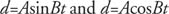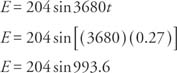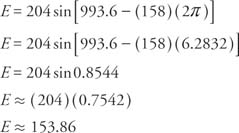## Simple Harmonic Motion

Circular functions representing periodic motion that satisfy the equationswhere d is an amount of displacement, A and B are constants determined by the specific motion, and t is a measurement of time are referred to as simple harmonic motion.

Example 1: If the instantaneous voltage in a current is given by the equation E = 204 sin 3680 t, where E is expressed in volts and t is expressed in seconds, find E if t = 0.27 seconds. Use 3.1416 for π.Since sin x = sin ( x2kπ ) and (993.6 + 2I÷2π) = 158 with a remainder of .8544,Example 2: The horizontal displacement (d) of the end of a pendulum is d = Ksin 2π t. Find K if d = 12 centimeters and t = 3.25 seconds.